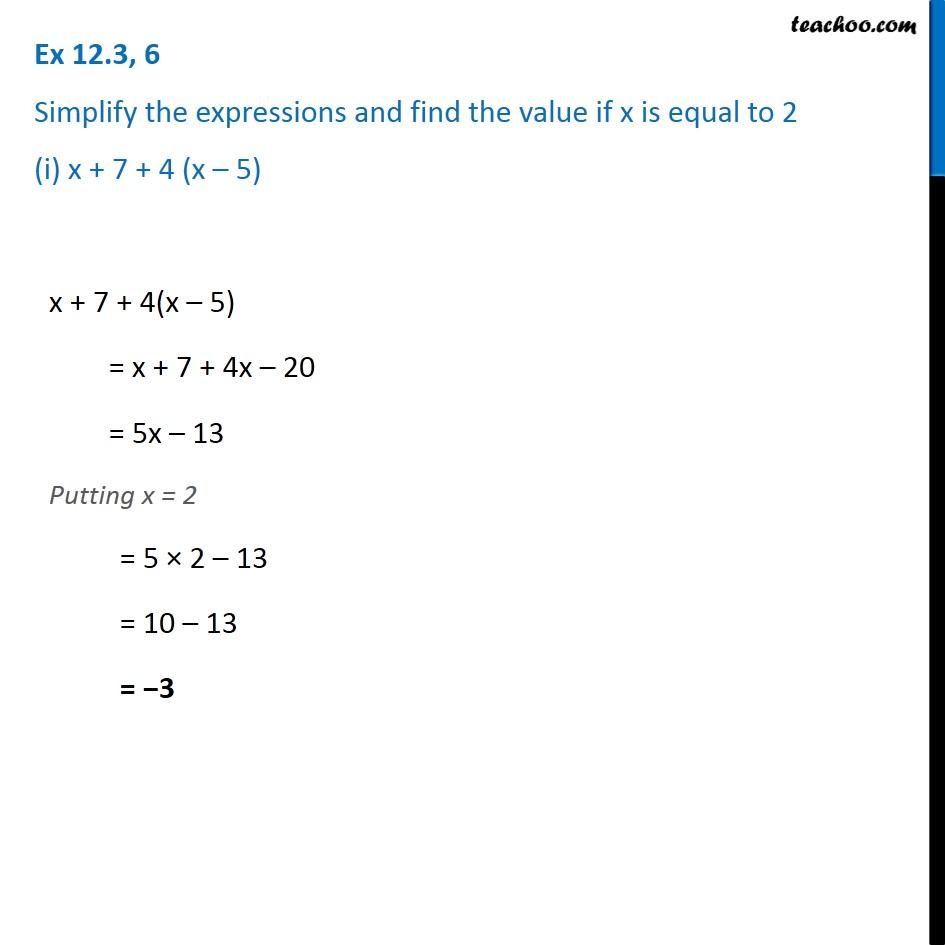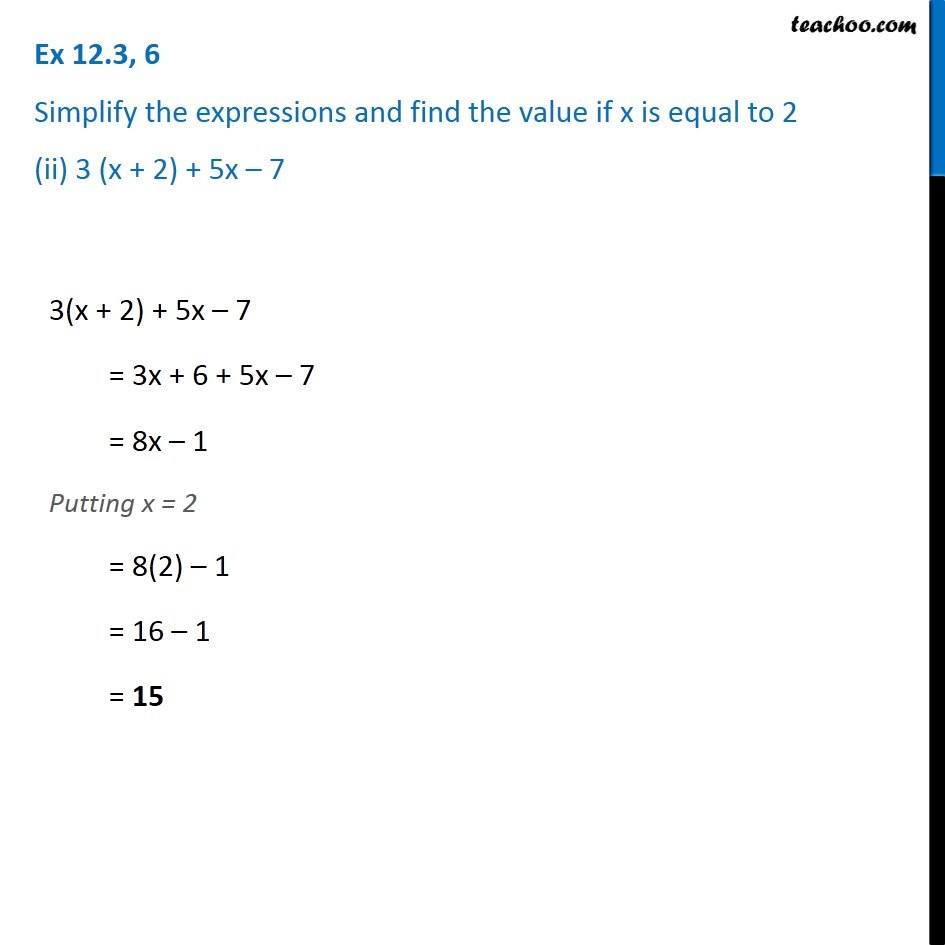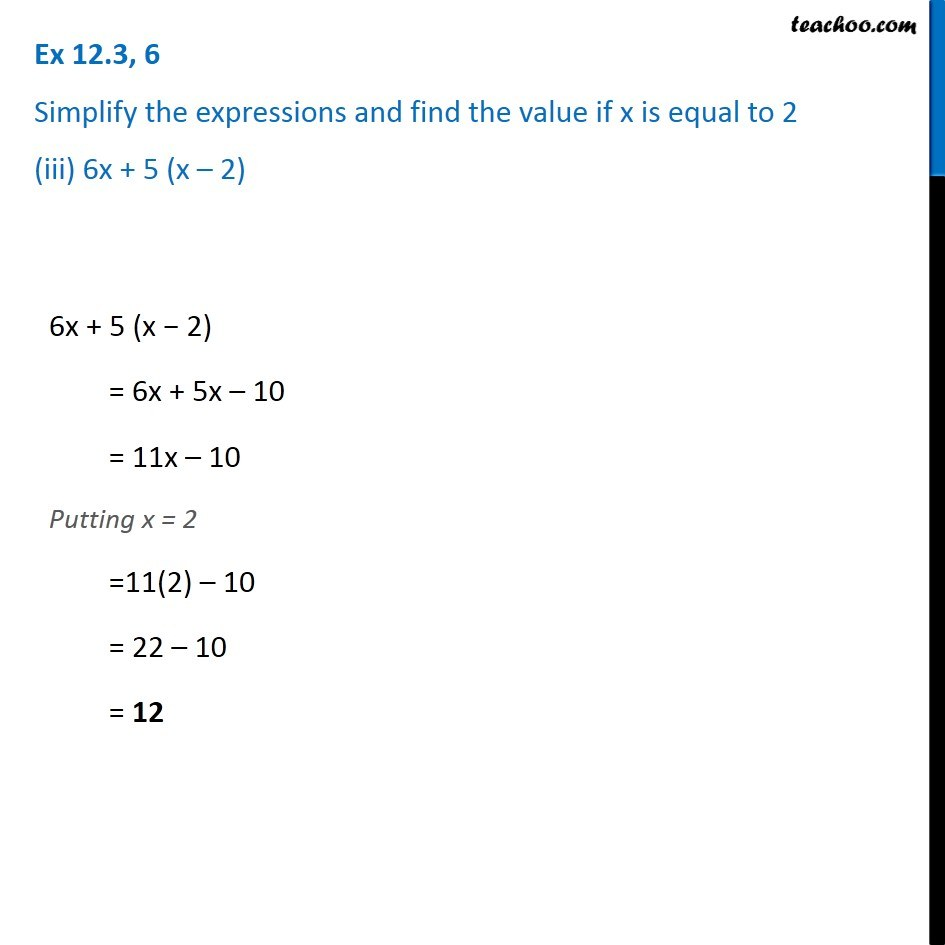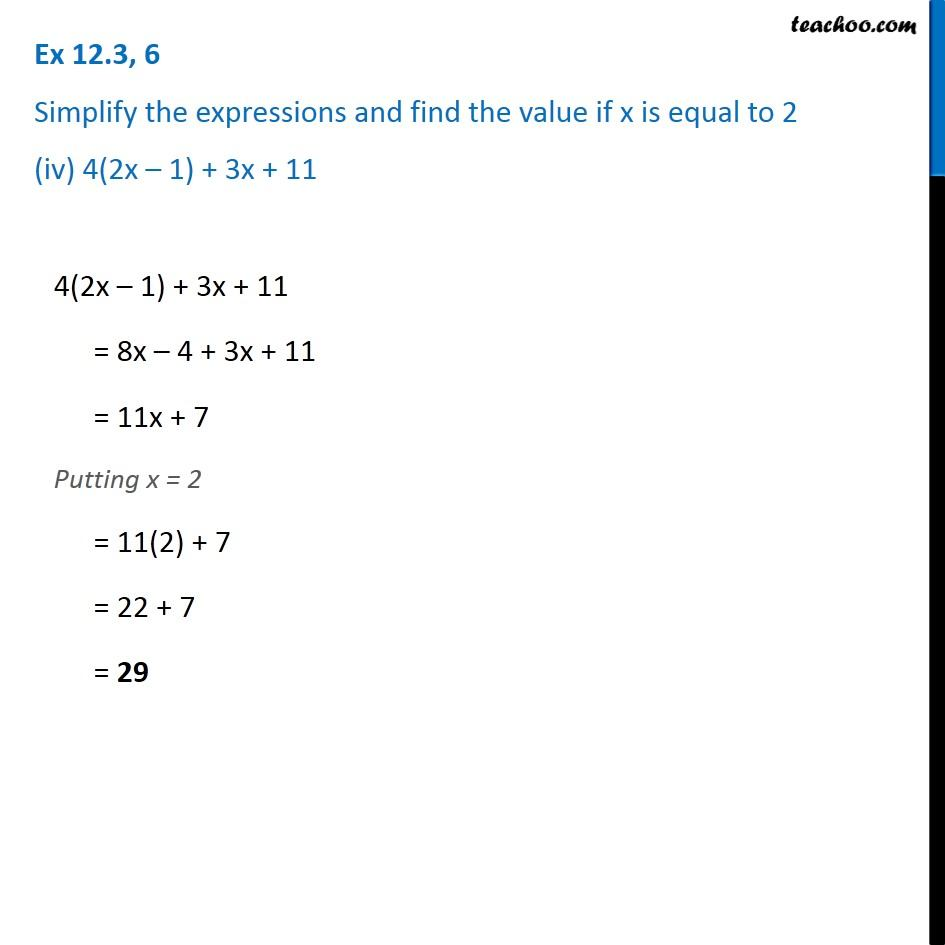Subscribe to our Youtube Channel - https://you.tube/teachoo

1. Chapter 12 Class 7 Algebraic Expressions
2. Serial order wise
3. Ex 12.3

Transcript

Ex 12.3, 6 Simplify the expressions and find the value if x is equal to 2 (i) x + 7 + 4 (x – 5) x + 7 + 4(x – 5) = x + 7 + 4x – 20 = 5x – 13 Putting x = 2 = 5 × 2 – 13 = 10 – 13 = −3 Ex 12.3, 6 Simplify the expressions and find the value if x is equal to 2 (ii) 3 (x + 2) + 5x – 7 3(x + 2) + 5x – 7 = 3x + 6 + 5x – 7 = 8x – 1 Putting x = 2 = 8(2) – 1 = 16 – 1 = 15 Ex 12.3, 6 Simplify the expressions and find the value if x is equal to 2 (iii) 6x + 5 (x – 2) 6x + 5 (x − 2) = 6x + 5x – 10 = 11x – 10 Putting x = 2 =11(2) – 10 = 22 – 10 = 12 Ex 12.3, 6 Simplify the expressions and find the value if x is equal to 2 (iv) 4(2x – 1) + 3x + 11 4(2x – 1) + 3x + 11 = 8x – 4 + 3x + 11 = 11x + 7 Putting x = 2 = 11(2) + 7 = 22 + 7 = 29

Ex 12.3